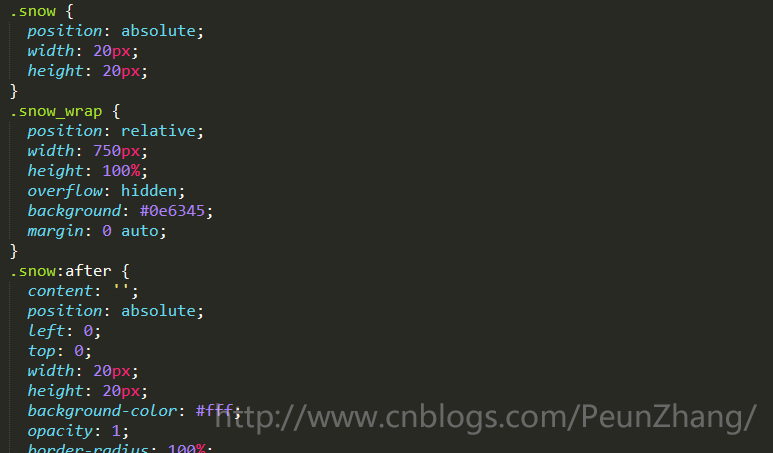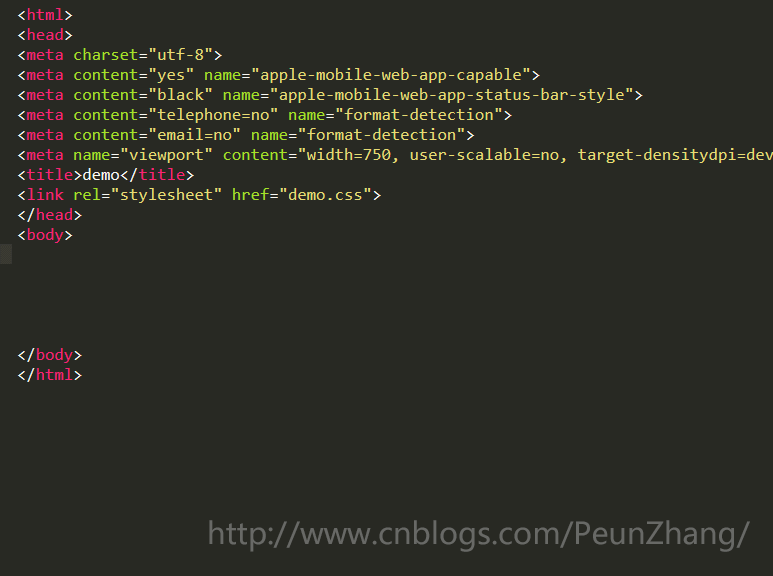# 如何使用less实现随机下雪动画详解

更新时间：2019年01月02日 11:44:57   作者：白树我要评论1.递归调用

```.snow(@n) when (@n > 0) {

fn()//生成雪花函数fn()

.snow((@n - 1));//再次执行函数fn()

}
.snow(60);//执行次数```

2.避免编译JavaScript 表达式

• 一些 LESS不认识的专有语法，可以在字符串前加上一个 ~，
• JavaScript 表达式在less 文件中使用，可以通过反引号的方式使用

```left: ~"`Math.round(Math.random() * @{windowWidth})`px";
animation: ~"snowani_@{n} `(-Math.random() * 4 + 8).toFixed(2)`s linear infinite";
transform: ~"scale(`(Math.random() * 0.7 + 0.5).toFixed(2)`)";```

```* {
padding: 0;
margin: 0;
}
html, body {
height: 100%;
}

//浏览器窗口宽度
@windowWidth: 750;
//雪花
.snow {
&_wrap{
position: relative;
width: ~"@{windowWidth}px";
height: 100%;
overflow: hidden;
background: rgba(14,99,69,1);
margin: 0 auto;
}
//雪花初始化大小
position: absolute;
width:20px;
height: 20px;
&:after{
content: '';
position: absolute;
left:0;
top:0;
width:20px;
height: 20px;
background-color:#fff;
opacity:1;
border-radius: 100%;
filter:blur(5px);//此处使用css3滤镜来画雪花
}
}
//随机雪花函数
.snow(@n) when (@n > 0) {
.snow_@{n}{
//水平方向上的位移
left: ~"`Math.round(Math.random() * @{windowWidth})`px";
//动画运行时间8~12秒，保证雪花有不同的移动速度
animation: ~"snowani_@{n} `(-Math.random() * 4 + 8).toFixed(2)`s linear infinite";
//动画提前出场时间，也就是垂直方向上位移
animation-delay: ~"`(-Math.random() * 8 + 0.2).toFixed(2)`s";
&:after{
//雪花大小随机，0.5~1.2
transform: ~"scale(`(Math.random() * 0.7 + 0.5).toFixed(2)`)";
}
}
@keyframes ~"snowani_@{n}" {
0%{
transform: translateY(0);
}
100%{
//垂直方向上高度，保证雪花有不同的移动速度
transform: ~"translateY(`Math.round(Math.random() * 200 + 1600)`px)";
}
}
.snow((@n - 1));
}
.snow(60);//生成雪花的数量````.snow_wrap>.snow.snow_\$*60`